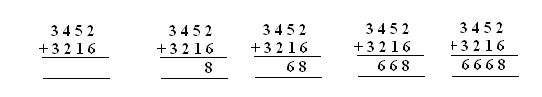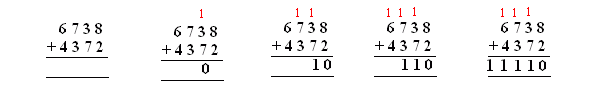Email us to get an instant 20% discount on highly effective K-12 Math & English kwizNET Programs!

#### Online Quiz (WorksheetABCD)

Questions Per Quiz = 2 4 6 8 10

 Method: Arrange the numbers one below the other. Add the numbers in ones place. Move on the tens, hundreds and thousands. Addition without carry Example: Add 3452 + 3216Start from ones place, 6 and 2 gives 8 In tens place 5 and 1 add up to 6. In hundreds place 4 and 2 give 6. In thousands place 3 and 3 give 6. Answer: 6668 Addition with carryIn ones place, sum is 10, 1 is carried to tens place. In tens place sum is 10, add the carry. This results in 11 with a carry 1 to hundreds place. In hundreds place sum is 10 , add the carry. This results in 11 with a carry 1 to thousands place. In ten thousands place, sum is 10 , add the carry, the result is 11. Answer: 11116 Directions: Add the following numbers. Also write at least ten examples of your own.